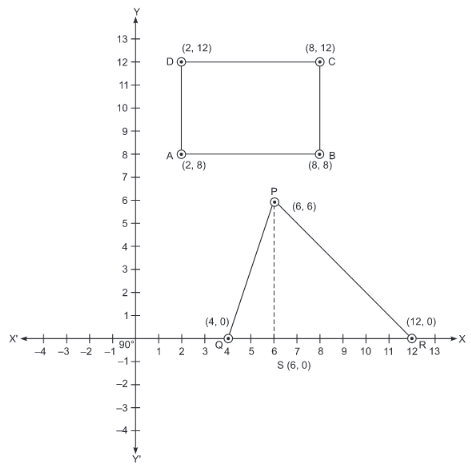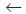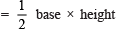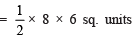Value Based Questions (VBQs) - Coordinate Geometry

# Value Based Questions (VBQs) - Coordinate Geometry Notes | Study Mathematics (Maths) Class 9 - Class 9

## Document Description: Value Based Questions (VBQs) - Coordinate Geometry for Class 9 2022 is part of Coordinate Geometry for Mathematics (Maths) Class 9 preparation. The notes and questions for Value Based Questions (VBQs) - Coordinate Geometry have been prepared according to the Class 9 exam syllabus. Information about Value Based Questions (VBQs) - Coordinate Geometry covers topics like and Value Based Questions (VBQs) - Coordinate Geometry Example, for Class 9 2022 Exam. Find important definitions, questions, notes, meanings, examples, exercises and tests below for Value Based Questions (VBQs) - Coordinate Geometry.

Introduction of Value Based Questions (VBQs) - Coordinate Geometry in English is available as part of our Mathematics (Maths) Class 9 for Class 9 & Value Based Questions (VBQs) - Coordinate Geometry in Hindi for Mathematics (Maths) Class 9 course. Download more important topics related with Coordinate Geometry, notes, lectures and mock test series for Class 9 Exam by signing up for free. Class 9: Value Based Questions (VBQs) - Coordinate Geometry Notes | Study Mathematics (Maths) Class 9 - Class 9
 1 Crore+ students have signed up on EduRev. Have you?

Question 1. KGB schools provide free education to girls students from weaker sections. The local body of a town want to open a KGB school in the town for which a rectangular plot ABCD, as shown in the following figure, is very suitable. But this plot belongs to Rati Ram. Rati Ram agrees to exchange it with triangular plot PQR as shown in the same figure. The co-ordinates of the vertices of both the plots are shown in the figure:(i) Compare the areas of both the plots.
(ii) Which mathematical concept is used in the above problem?
(iii) By opening KGB school in the town, which value is depicted by the local body?
Solution. (i) For rectangle ABCD:
AB = 8 – 2 = 6 unitsLength
AD = 12 – 8 = 4 unitsBreadth
∴ Area of rectangle ABCD = AB × AD
= 6 units × 4 units
= 24 sq. units.

For triangle PQR:
QR = 12 – 4 = 8 unitsBase
SP = 6 – 0 = 6 unitsHeight
∴ Area of DPQR= 24 sq. units.

⇒  [area of rect. ABCD] = [area of ΔPQR]

(ii) Co-ordinate Geometry.
(iii) Community or social upliftment.

The document Value Based Questions (VBQs) - Coordinate Geometry Notes | Study Mathematics (Maths) Class 9 - Class 9 is a part of the Class 9 Course Mathematics (Maths) Class 9.
All you need of Class 9 at this link: Class 9

## Mathematics (Maths) Class 9

84 videos|352 docs|109 tests
 Use Code STAYHOME200 and get INR 200 additional OFF

## Mathematics (Maths) Class 9

84 videos|352 docs|109 tests

Track your progress, build streaks, highlight & save important lessons and more!

,

,

,

,

,

,

,

,

,

,

,

,

,

,

,

,

,

,

,

,

,

;